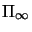Next: From Affine to Euclidean Up: From Projective to Affine Previous: The Need for Affine

## Defining an Affine Restriction

As we have seen in chapter 2, affine transformations leave the line at infinity invariant. In fact the group of affine transformations is just the subgroup of the projective group that maps this line (or plane) onto itself. Any line can be chosen to be fixed, and then the projective subgroup that leaves this line fixed defines a valid affine space.

Usually we want to interpret the fixed line as being the effective line at infinity of a hidden affine space. For example, this happens when a 3D plane is projected to an image under perspective projection. The line at infinity of the 3D plane is projected to a corresponding horizon line'', which is not usually at infinity in the image. Once this horizon line has been identified and fixed, the affine geometry defined is the one pertaining to the original 3D plane, and not to the camera image plane. To implement this, we just need to find a change of coordinates that maps this line to the one with dual coordinate vector (0,0,1).

Sometimes (as with the real horizon) this horizon line is actually visible in the image. If not, we might use some prior knowledge about the scene to compute its position. For instance, if we know that two 3D lines are parallel, their intersection directly gives the image of one point at infinity. Another useful piece of information is the 3D distance ratio of three aligned points. The cross ratio of the ideal point on the line through these is the same as the distance ratio in affine space, so the image of the ideal point can be computed from (3.2), given the affine ratio and the projections of the three points.

Exercise 4.1   : You observe the perspective image of a plane parallelogram. Derive an algebraic expression for the plane's horizon (i.e. the projection of the line at infinity) in terms of the vertex projections a,b,c,d.

Exercise 4.2   : You observe the image of three parallel, equally spaced, coplanar lines. Explain how to compute the horizon line of the plane.

Once the ideal line (or plane) has been located in projective space, computing affine information is straightforward. Stating thatshould have equation t=0 yields 2 independent linear constraints on the last line of the permissible collineation matrices W (3 in 3D).Next: From Affine to Euclidean Up: From Projective to Affine Previous: The Need for Affine
Bill Triggs
1998-11-13